Next:
Hermitian Observables Up: Quantum Mechanics: Two-state Systems

#### Quantum States

Generally, the quantum state of a physical system is specified by a non-zero vector in a Hilbert space over the complex numbers (call it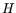), with the understanding that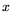andspecify the same quantum state, whereis inand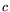is a non-zero complex number. In other words, the set of quantum states is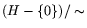, where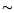is the equivalence relation defined byif and only iffor some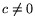. Sometimes I will abuse language, and say the state vector of the system is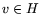'' instead of saying the state of the system is specified by.''

The state of our spinning electron is therefore specified by giving a ratio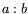of two complex numbers (since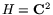), not both zero. Mathematically, this is just the complex projective line. The correspondence between points on the complex projective line and points on the Riemann sphere is well-known; I will make use of it in a moment. 3

Physically, we want to picture the electron as a little ball, spinning about some axis. It has angular momentum. In more detail, the angular momentum vector is a vector pointing along the axis of rotation, with length proportional to the speed of rotation. This defines the vector up to sign. The sign ambiguity is resolved, conventionally, by the right hand rule of thumb: if you curl the fingers of your right hand in the direction of rotation, the thumb points in the direction of the angular momentum vector.

Classically, the electron could be spinning at any speed, so its angular momentum could have any magnitude. In quantum mechanics, the angular momentum is quantized: its magnitude (measured along the axis of rotation) must be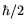for any spinparticle. (Note:, whereof course is Planck's constant.)

The quantization of angular momentum, although inexplicable classically, is easy enough to picture: we just stipulate that our spinning ball must be spinning at a particular rate. So we should be able to specify the spin state of the electron just by giving a direction in 3-space, or equivalently, by picking a point on the sphere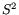. But I just noted that the set of states is isomorphic'' to the Riemann sphere. So everything fits.Next:
Hermitian Observables Up: Quantum Mechanics: Two-state Systems

© 2001 Michael Weiss